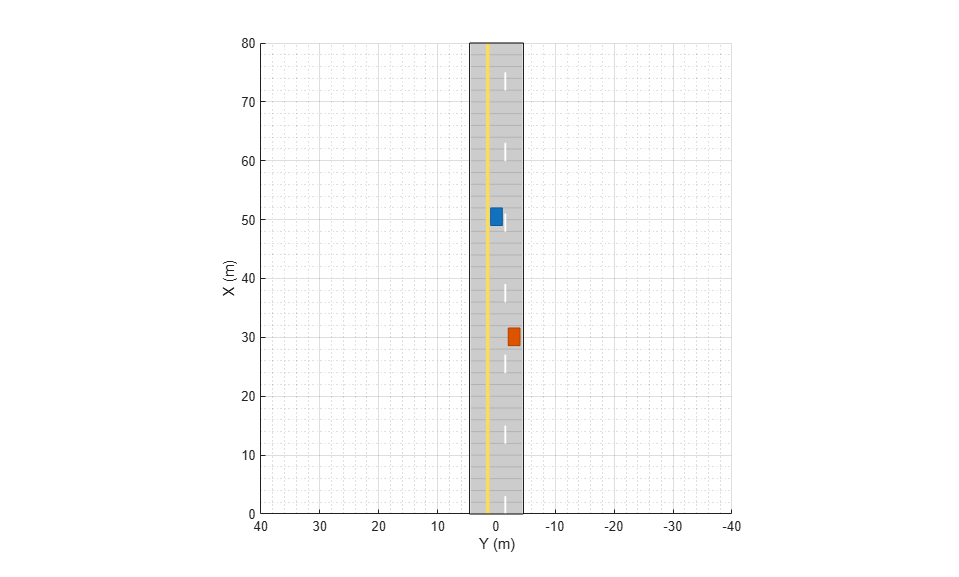# currentLane

Get current lane of actor

## Syntax

``cl = currentLane(ac)``
``[cl,numlanes] = currentLane(ac)``

## Description

````cl = currentLane(ac)` returns the current lane, `cl`, of an actor, `ac`.```

example

````[cl,numlanes] = currentLane(ac)` also returns the number of road lanes, `numlanes`.```

## Examples

collapse all

Obtain the current lane boundaries of cars during a driving scenario simulation.

Create a driving scenario containing a straight, three-lane road.

```scenario = drivingScenario; roadCenters = [0 0; 80 0]; road(scenario,roadCenters,'Lanes',lanespec([1 2],'Width',3)); ```

Add an ego vehicle moving at 20 meters per second and a target vehicle moving at 10 meters per second.

```ego = vehicle(scenario,'ClassID',1,'Position',[5 0 0], ... 'Length',3,'Width',2,'Height',1.6); smoothTrajectory(ego,[1 0 0; 20 0 0; 30 0 0;50 0 0],20); target = vehicle(scenario,'ClassID',1,'Position',[5 0 0], ... 'Length',3,'Width',2,'Height',1.6); smoothTrajectory(target,[5 -3 0; 20 -3 0; 30 -3 0;50 -3 0],10); ```

Plot the scenario.

```plot(scenario) ```Run the simulation loop.

```while advance(scenario) [cl1,numlanes] = currentLane(ego); [cl2,numlanes] = currentLane(target); end ```Display the current lane of each vehicle.

```disp(cl1) disp(cl2) ```
``` 2 3 ```

## Input Arguments

collapse all

Actor belonging to a `drivingScenario` object, specified as an `Actor` or `Vehicle` object. To create these objects, use the `actor` and `vehicle` functions, respectively.

## Output Arguments

collapse all

Current lane of the actor, returned as a positive integer. Lanes are numbered from left to right, relative to the actor, starting from 1. When the actor is not on a road or is on a road without any lanes specified, `cl` is returned as empty, `[]`.

Number of lanes on the road that the actor is traveling on, returned as a positive integer. When the actor is not on a road or is on a road without any lanes specified, `numlanes` is returned as empty, `[]`.

## Version History

Introduced in R2018a